2001 OASDI Trustees Report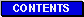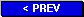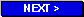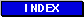### B. HISTORY OF ACTUARIAL BALANCE ESTIMATES

This appendix chronicles the history of the principal summary measure of long-range actuarial status, namely the actuarial balance, since 1983. The 1983 report was the last report for which the actuarial balance was positive. Actuarial balance is defined in detail in chapter IV, Actuarial Estimates. Conceptually, the two basic components of actuarial balance are the summarized income rate and the summarized cost rate. Both rates are expressed as percentages of taxable payroll. For any given period, the actuarial balance is the difference between the present value of tax income for the period, and the present value of the outgo for the period, each divided by the present value of taxable payroll for all years in the period. Also included in the calculation of the actuarial balance are:

• The amount of the trust fund balances on hand at the beginning of the valuation period, as shown in the reports for 1988 and later, and

• The present value of a target trust fund balance equal to 100 percent of the amount of annual outgo to be reached and maintained by the end of the valuation period, as shown in the reports for 1991 and later.

It should be noted that the current method of calculating the actuarial balance based on present values, though used prior to the 1973 Annual Report, was not used for the annual reports of 1973-87. Instead, a simpler method that approximates the results of the present-value approach, called the average-cost method, was used during that period. Under the average-cost method, the sum of the annual cost rates (which are expressed as percentages of taxable payroll) over the 75-year projection period was divided by the total number of years, 75, to obtain the average cost rate per year. The average income rate was similarly calculated, and the difference between the average income rate and the average cost rate was called the actuarial balance.

In 1973, when the average-cost method was first used, the long-range financing of the program was more nearly on a pay-as-you-go basis. Also, based on the long-range economic and demographic assumptions then being used, the annual rate of growth in taxable payroll was about the same as the annual rate at which the trust funds earned interest. In either situation (i.e., pay-as-you-go financing, where the annual income rate is the same as the annual cost rate, or an annual rate of growth in taxable payroll equal to the annual interest rate), the average-cost method produces the same result as the present-value method. However, by 1988, neither of these situations still existed.

As a result of legislation enacted in 1977 and in 1983, substantial increases in the trust funds were estimated to occur well into the 21st century, so that the program was partially advance funded, rather than being funded on a pay-as-you-go basis. Also, because of reductions in long-range fertility rates and average real-wage growth that were assumed in the annual reports over the period 1973-87, the annual rate of growth in taxable earnings assumed for the long range became significantly lower than the assumed interest rate. Therefore, during the period 1973-87, the results of the average-cost method and the present-value method began to diverge, and by 1988 they were quite different. While the average-cost method still accounted for most of the effects of the assumed interest rate, it no longer accounted for all of the interest effects. The present-value method, of course, does account for the full effect of the assumed interest rates. So, in 1988, the present-value method of calculating the actuarial balance was reintroduced.

A positive actuarial balance indicates that estimated income is more than sufficient to meet estimated trust fund obligations for the period as a whole. A negative actuarial balance indicates that estimated income is insufficient to meet estimated trust fund obligations for the entire period. An actuarial balance of zero indicates that the estimated income exactly matches estimated trust fund obligations for the period.

Table VI.B1 shows the estimated OASDI actuarial balances, as well as the summarized income and cost rates, for the annual reports 1983-2000, along with the estimates for the current report. The values shown are based on the intermediate alternative II assumptions, or alternative II-B for years prior to 1991.

Table VI.B1.- Long-Range OASDI Actuarial Balances1 as Shown
in the Trustees Reports for 1983-2001
[As a percentage of taxable payroll]
Year of report
Summarized
income rate
Summarized
cost rate
Actuarial
balance
Change from
previous year
1983
12.87
12.84
+0.02
+1.84
1984
12.90
12.95
-.06
-.08
1985
12.94
13.35
-.41
-.35

1986
12.96
13.40
-.44
-.03
1987
12.89
13.51
-.62
-.18
1988
12.94
13.52
-.58
+.04
1989
13.02
13.72
-.70
-.13
1990
13.04
13.95
-.91
-.21

1991
13.11
14.19
-1.08
-.17
1992
13.16
14.63
-1.46
-.38
1993
13.21
14.67
-1.46
(2)
1994
13.24
15.37
-2.13
-.66
1995
13.27
15.44
-2.17
-.04

1996
13.33
15.52
-2.19
-.02
1997
13.37
15.60
-2.23
-.03
1998
13.45
15.64
-2.19
+.04
1999
13.49
15.56
-2.07
+.12
2000
13.51
15.40
-1.89
+.17

2001
13.58
15.44
-1.86
+.03
 1 Values shown are based on the intermediate alternative II assumptions for 1991-2001, and on the intermediate alternative II-B assumptions for 1982-90. 2 Between -0.005 and 0.005 percent of taxable payroll.

Note: Totals do not necessarily equal the sums of rounded components.

For several of the years included in the table, significant legislative changes or definitional changes affected the estimated actuarial balance. The Social Security Amendments of 1983 accounted for the largest single change in recent history. The actuarial balance of -1.82 for the 1982 report improved to +0.02 for the 1983 report. In 1985, the estimated actuarial balance changed largely because of an adjustment made to the method for estimating the age distribution of immigrants.

Rebenchmarking of the National Income and Product Accounts and changes in demographic assumptions contributed to the change in the actuarial balance for 1987. Various changes in assumptions and methods for the 1988 report had roughly offsetting effects on the actuarial balance. In 1989 and 1990, changes in economic assumptions accounted for most of the changes in the estimated actuarial balance. In 1991, the effect of legislation, changes in economic assumptions, and the introduction of the cost of reaching and maintaining an ending trust fund target combined to produce the change in the actuarial balance. In 1992, changes in disability assumptions and the method for projecting average benefit levels accounted for most of the change in the actuarial balance. In 1993, numerous small changes in assumptions and methods had offsetting effects on the actuarial balance. In 1994, changes in the real-wage assumptions, disability rates, and the earnings sample used for projecting average benefit levels accounted for most of the change in the actuarial balance. In 1995, numerous small changes had largely offsetting effects on the actuarial balance, including a substantial reallocation of the payroll tax rate, which reduced the OASI actuarial balance, but increased the DI actuarial balance. In 1996, a change in the method of projecting dually-entitled beneficiaries produced a large increase in the actuarial balance, which almost totally offset decreases produced by changes in the valuation period and in the economic and demographic assumptions. Various changes in assumptions and methods for the 1997 report had roughly offsetting effects on the actuarial balance. In 1998, increases caused by changes in the economic assumptions, although partially offset by decreases produced by changes in the valuation period and in the demographic assumptions, accounted for most of the changes in the estimated actuarial balance. In 1999, increases caused by changes in the economic assumptions related to improvements in the CPI by the Bureau of Labor Statistics accounted for most of the changes in the estimated actuarial balance. For the 2000 report, changes in the actuarial balance resulted from changes in economic assumptions and methodology; however, these increases in the balance were partially offset by reductions caused by the change in valuation period and changes in demographic assumptions. Changes affecting the actuarial balance shown for the 2001 report are described in section IV.B.7.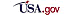Privacy Policy | Website Policies & Other Important Information  | Site Map  | Actuarial Publications 3/19/2001